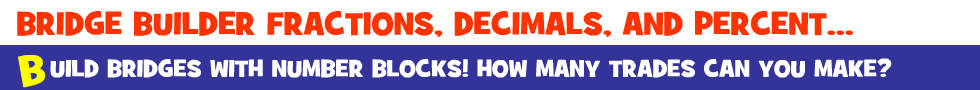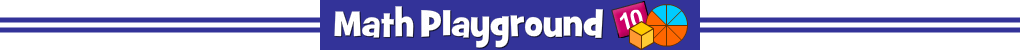Build bridges with the fewest number of blocks.
Combine expensive smaller blocks and trade them for cost-saving larger blocks.
The goal is to keep the cost as low as possible.
Check the value of the new block, select smaller blocks to trade, then tap the Trade button.
You may only trade blocks that are in the same row.
Bridge Builder Decimals - Learning Connections
Essential Skills
Mental Math - compare fractions, decimals, percents

Common Core Connection for Grades 4 and 5
Compare two fractions with different numerators and different denominators.
Understand decimal notation for fractions, and compare decimal fractions.
Recognize and generate simple equivalent fractions.
Develop an understanding of fractions and decimals as numbers.
More Math Games to Play